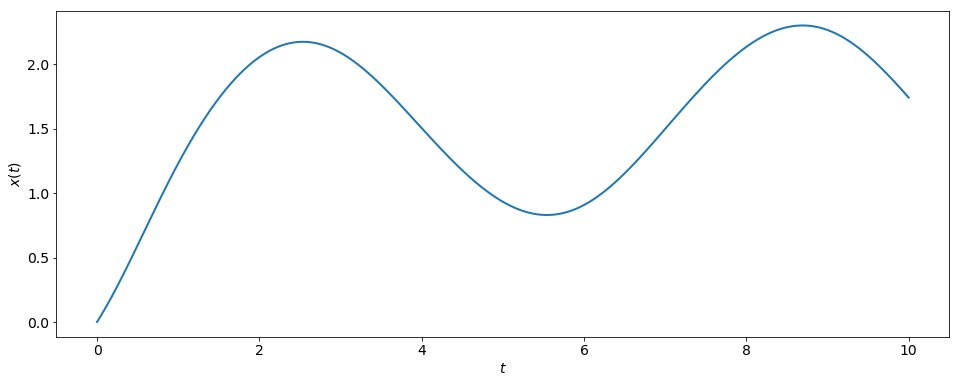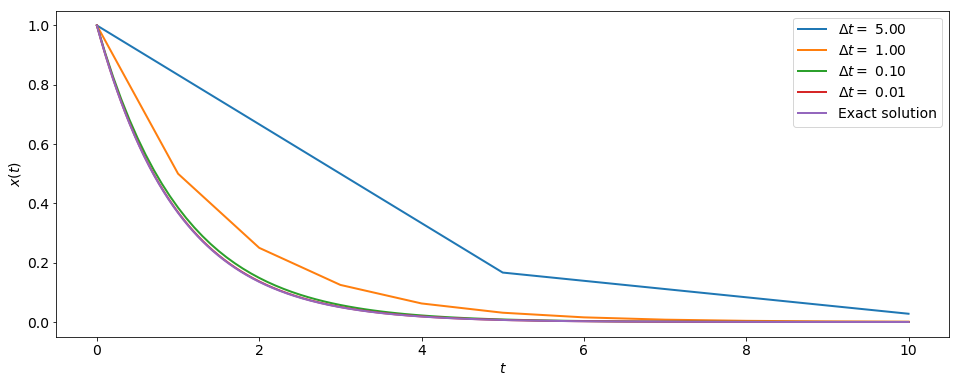# Implicit Euler Method¶

### Modules - Ordinary Differential Equations¶

Last edited: March 11th 2018

We have already seen one method for solving ordinary differential equations (ODEs) of the form $$\frac{d}{dx}x(t) = g(x(t),t),~~~x(t_0)=x_0,$$ namely the explicit Euler method, or simply Euler's method. There one first discretizes time using $$t_n = t_0 + n \Delta t \, n=0,1...,N,$$ with $$\Delta t = \frac{t_N-t_0}{N}.$$ Then one approximates the time derivative of $x(t)$ at time $t_n$ using the forward difference approximation, $$\frac{dx}{dt}\Big|_{t=t_n}\approx \frac{x(t_{n+1})-x(t_n)}{\Delta t},~~~~~\Delta t>0.$$ This is then inserted into the ODE, evaluating $g(x,t)$ at the point $x(t_n)$ and time $t_n$.

In the implicit Euler method (or Backward Euler method), one evaluates $g(x,t)$ using the new timestep $t_{n+1}$, and hence also using $x(t_{n+1})$, the value of which is unknown! We then get the following formula $$x_{n+1}= x_n +\Delta t \cdot g(x_{n+1},t_{n+1}),$$ were we have introduced the notation $x_n \equiv x(t_n)$.

We quickly realize that this might not be as simple as for the explicit Euler method, were one gets a simple iterative formula. This is, however, not the case here, since we will have to find the root of the function $$f(x_{n+1}) = x_{n+1}- x_n -\Delta t \cdot g(x_{n+1},t_{n+1})$$ in order to determine $x_n$. Let's illustrate this with an example.

Example:

We want to solve $$\frac{d}{dt}x(t)=\cos(x(t))+\sin(t), \,x(t_0=0)=0,$$ using the implicit Euler method. This yields $$x_{n+1} = x_n + \Delta t\left(\cos(x_{n+1})+\sin(t_{n+1})\right),$$ and we want to find the values $x_{n+1}$ such that $$f(x_{n+1}) = x_{n+1} - x_n - \Delta t\left(\cos(x_{n+1})+\sin(t_{n+1})\right) = 0.$$ The code needed to solve this problem is implemented below, and the result plotted. In order to compare with the explicit Euler method, we'll use the same step size. Since we need to find the root of a function, we first implement Newton's method.

In :
%matplotlib inline
import numpy as np
import matplotlib.pyplot as plt

# Set common figure parameters
newparams = {'figure.figsize': (16, 6), 'axes.grid': True,
'lines.linewidth': 1.5, 'lines.linewidth': 2,
'font.size': 14}
plt.rcParams.update(newparams)

In :
def newton(x_old, t_new, dt, precision=1e-12):
""" Takes values x_old and t_new, and finds the root of the
function f(x_new), returning x_new. """

# initial guess:
x_new = x_old
f = func(x_new, x_old, t_new, dt)
dfdx = dfuncdx(x_new, dt)

while abs(f/dfdx) > precision:
x_new = x_new - f/dfdx
f = func(x_new, x_old, t_new, dt)
dfdx = dfuncdx(x_new, dt)

return x_new

def func(x_new, x_old, t_new, dt):
""" The function f(x) we want the root of."""
return x_new - x_old - dt*(np.cos(x_new) + np.sin(t_new))

def dfuncdx(x_new, dt):
""" The derivative of f(x) with respect to x_new."""
return 1+dt*np.sin(x_new)

In :
dt = 0.001  # step size
t_max = 10  # maximum time
N = int(t_max/dt)

t = np.linspace(0, t_max, N+1)
x = np.zeros(N+1)

# set initial value
x = 0

for n in range(N):
x[n+1] = newton(x[n], t[n+1], dt)

print(r'x_N = %f' % x[-1])

plt.plot(t,x)
plt.xlabel(r'$t$')
plt.ylabel(r'$x(t)$')
plt.grid();

x_N = 1.742352We see that this is very similar to the result obtained using the explicit Euler method.

#### Accuracy and Stability:¶

Just like the explicit Euler method, the implicit method is a first-order method. That means that the global truncation error is proportional to the step size $\Delta t$. I.e., if one divides $\Delta t$ by two, the truncation error will also be halved.

Let's also look at the stability of the implicit Euler method, by considering the ODE $$\frac{dx(t)}{dt} = -x, ~~\mathrm{with}~~ x(0) = 1.$$ The exact soluton is $x(t) = e^{-t}$. For the explicit Euler method we found that the method only was stable for $0<\Delta t<1$. For the implicit method, however, we get: \begin{align} x_{n+1} &= x_n - \Delta t \cdot x_{n+1} \\ x_{n+1} &= \frac{x_n}{1+\Delta t}\leq x_n\, \mathrm{for} \, \Delta t\geq 0. \end{align} Hence we see that the implicit Euler method can be unconditionally stable for certain ODEs, as it is for the one above. This is however not always the case!

Remember that even thought the method is stable, the accuracy might not be good. To illustrate this, we will solve the example ODE above for different values of $\Delta t$ and compare with the exact solution. Note that we don't need a root solver in this case, since solving for is trivial in the case considered here.

In :
dts = np.array([5, 1, 0.1, 0.01])
t_max = 10

plt.figure()
for i, dt in enumerate(dts):
N = int(t_max/dt)
t = np.linspace(0, t_max, N+1)
x = np.zeros(N+1)
x = 1

for n in range(N):
x[n+1] = x[n]/(1+dt)

# Plot the solution
plt.plot(t,x)

# Plot exact solution
plt.plot(t, np.exp(-t))
plt.xlabel(r'$t$')
plt.ylabel(r'$x(t)$')
plt.legend([r'$\Delta t=$ %0.2f' % dts, r'$\Delta t=$ %0.2f' % dts, r'$\Delta t=$ %0.2f' % dts,
r'$\Delta t=$ %0.2f' % dts, r'Exact solution'])
plt.grid();We see that all solutions are stable, and that the accuracy increases with decreasing $\Delta t$.